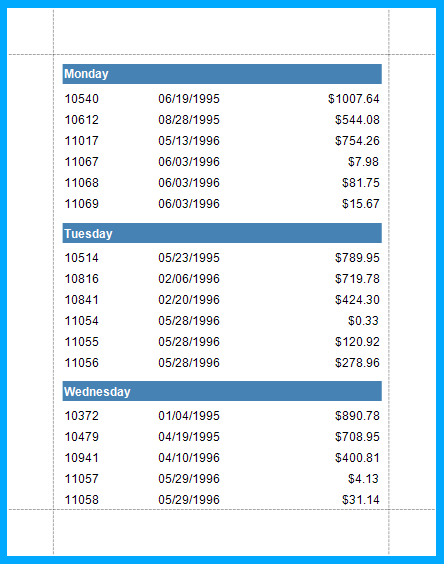21.2 (EAP/Beta)
21.1
20.2
20.1
19.2
19.1
18.2
18.1
17.2

# Group Data by Days of the Week (Runtime Sample)

This example illustrates how to group a report by a time span value in code. The report created in this example is grouped and sorted by the days of the week:The report created in this example is bound to the “Orders” table of the sample Northwind database (the nwind.mdb file located in the directory where you installed DevExpress demos).

To sort records by days of the week at runtime, do the following.

1. Create a calculated field with the CalculatedField.FieldType property set to String. Add a GroupHeader band, and add a grouping criteria (with the GroupField.FieldName property set to the created calculated field) to its GroupHeaderBand.GroupFields collection.
2. Then, drop the calculated field from the Field List onto the GroupHeader1 band for the XRLabel control (named hdrLabel) bound to the field to be automatically created.
3. Handle the calculated field’s CalculatedField.GetValue and the hdrLabel‘s XRControl.BeforePrint event handlers in the following way.
``````using System;
using System.Data;
using System.Windows.Forms;
using DevExpress.XtraReports.UI;
// ...

private void calculatedField1_GetValue(object sender, GetValueEventArgs e) {
object columnValue = e.GetColumnValue("OrderDate");
e.Value = (int)((DateTime)columnValue).DayOfWeek;
}

private void hdrLabel_BeforePrint(object sender, System.Drawing.Printing.PrintEventArgs e) {
hdrLabel.Text = ((DayOfWeek)this.GetCurrentColumnValue(this.calculatedField1.Name)).ToString();
}
``````